# Portenta Pin Mapping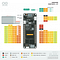Figure 1. Arduino Portenta H7 Pinout.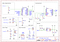Figure 2. MKR Sensor Shield Schematic.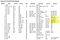Figure 3. Magpie Drone Portenta Pin Map.

# Files to be Changed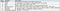Figure 4. Understanding the Portenta MCU Model Number.
`//PORTJ — D(AF, TIMx)#define DEF_TIM_AF__PJ6__TCH_TIM8_CH2 D(3, 8)#define DEF_TIM_AF__PJ8__TCH_TIM8_CH1 D(3, 8)#define DEF_TIM_AF__PJ9__TCH_TIM1_CH3 D(1, 1)#define DEF_TIM_AF__PJ10__TCH_TIM8_CH2 D(3, 8)#define DEF_TIM_AF__PJ11__TCH_TIM1_CH2 D(1, 1)`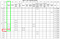Figure 6. Port J Alternate Functions Table — STM32H747 Data Sheet.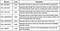Figure 7. DFU Requests (credit).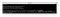Figure 8. Using dfu-util to look for connected devices.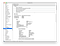Figure 9. Arduino Portenta VID and PID.
`var usbDevices = {filters: [{ ‘vendorId’: 1155, ‘productId’: 57105 },{ ‘vendorId’: 10473, ‘productId’: 393 },{ ‘vendorId’: 9025, ‘productId’: 859 } /* Arduino / H7 */]};Figure 10. Adding the Arduino Portenta VID and PID to BF Configurator.`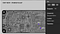Figure 11. Location of TP3 (BOOT) on the Portenta H7.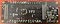Figure 12. Location of TP3 (bottom view).

# Appendix A — File Change Notes

`SCK_PIN = PF10IO0_PIN = PD11IO1_PIN = PD12IO2_PIN = PF7IO3_PIN = PD13CS_PIN = PG6`
`SPI2_SCK_PIN = PI1 (note the pin numbering is incorrect in Figure 1.)SPI2_MISO_PIN = PC2SPI2_MOSI_PIN = PC3SPI2_NSS_PIN = PI0GYRO_1_EXTI_PIN = PK1`
`I2C3_SCL = PH7I2C3_SDA = PH8`
`DEF_TIM( TIM5, CH1, PA8, TIM_USE_MOTOR, 0, 0, 0 ), // M1`
`typedef struct pllConfig_s {uint16_t clockMhz;uint8_t m;uint16_t n;uint8_t p;uint8_t q;uint8_t r;uint32_t vos;} pllConfig_t;               Figure 9. The PLL1 Config Structure.`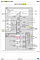Figure 10. PLL1 Config — STM32H747 Reference Manual (credit).
`// 27MHz for Arduino Portenta H7pllConfig_t pll1ConfigPortentaH7 = {.clockMhz = 27,.m = 4,.n = 27,.p = 2,.q = 8,.r = 5,.vos = PWR_REGULATOR_VOLTAGE_SCALE0};         Figure 11. Portenta H7 PLL1 Configuration.`

# Appendix B — Futher Details…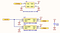Figure 12. Portenta External Oscillators (credit).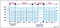Figure 13. Quad SPI Data (credit).
`my @ports = (‘A’, ‘B’, ‘C’, ‘D’, ‘E’, ‘F’, ‘G’, ‘H’, ‘I’, ‘J’, ‘K’);`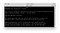Figure 14. Determing if Perl is installed.

Reefwing Software · iOS & Android Development · Robotics · #followback #iOS #Android #developer #indiedev #robotics #startup #arduino #raspberrypi

## More from David Such

Reefwing Software · iOS & Android Development · Robotics · #followback #iOS #Android #developer #indiedev #robotics #startup #arduino #raspberrypi